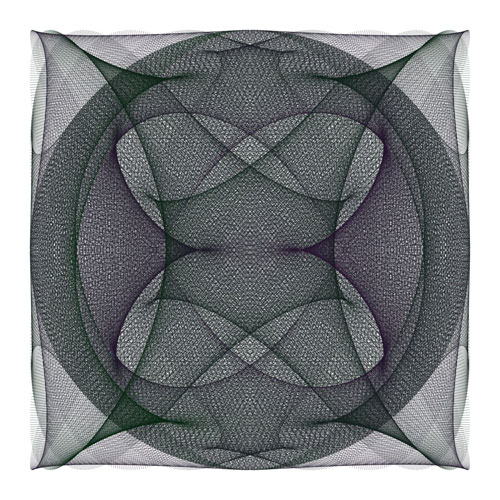Math Central - mathcentral.uregina.caThe art of Hamid Naderi YeganehResource RoomI am a student of mathematics at University of Qom. I won gold medal at 38th Iranian Mathematical Society's Competition (May 2014). I live in Iran. I like to create beautiful images by basic mathematical concepts. You can see some of my works at: https://mathematics.culturalspot.org and on the American Mathematical Society site.This image shows 4,000 line segments. For each $i = 1, 2, 3, \cdot \cdot \cdot, 4000$ the endpoints of the i-th line segment are: $\left(\sin \left( \frac{14 \pi\; i}{4000} \right),\; \cos \left( \frac{22 \pi\; i}{4000} \right) \right)$ and $\left( \sin \left( \frac{34 \pi\; i}{4000} \right),\; \cos \left( \frac{34 \pi\; i}{4000} \right) \right).$
 Math Central is supported by the University of Regina and the Pacific Institure for the Mathematical Sciences.about math central :: site map :: links :: notre site français